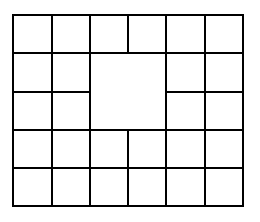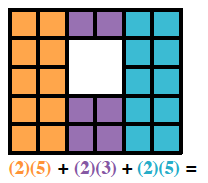### Home > CC1 > Chapter 4 > Lesson 4.1.2 > Problem4-16

4-16.

Look at the figure formed by square tiles below. How can you find out how many small squares there are in this diagram without counting each one? Think about this as you answer the questions below. Homework Help ✎1. Write and simplify an expression involving addition to count the number of small squares.

• Try grouping the square tiles into columns and writing an expression to represent them.$26$ squares

1. Write and simplify an expression involving subtraction to count the number of small squares.

• In order to use subtraction, you will need to first find how many squares there would be if the blank section
in the middle were actually squares.